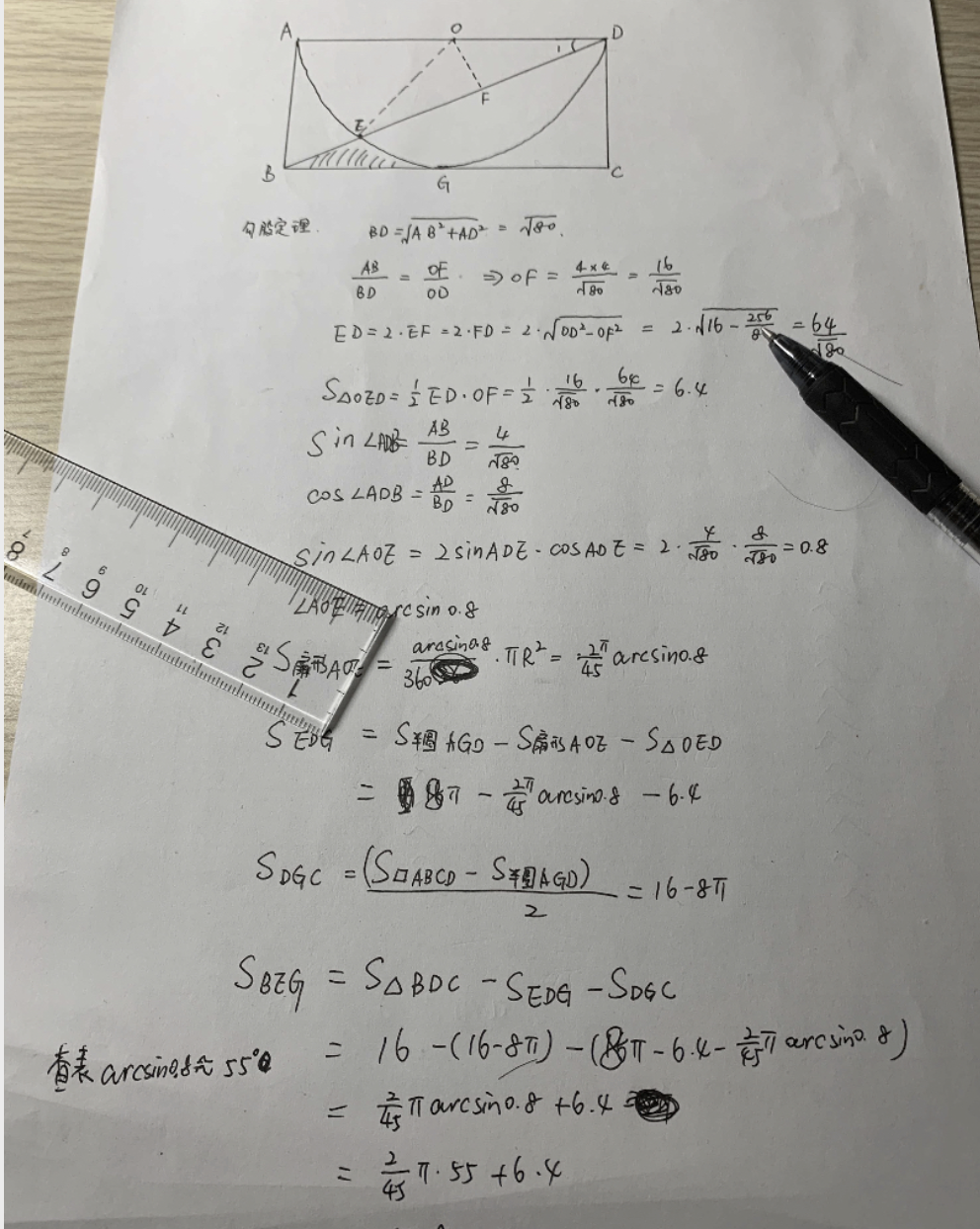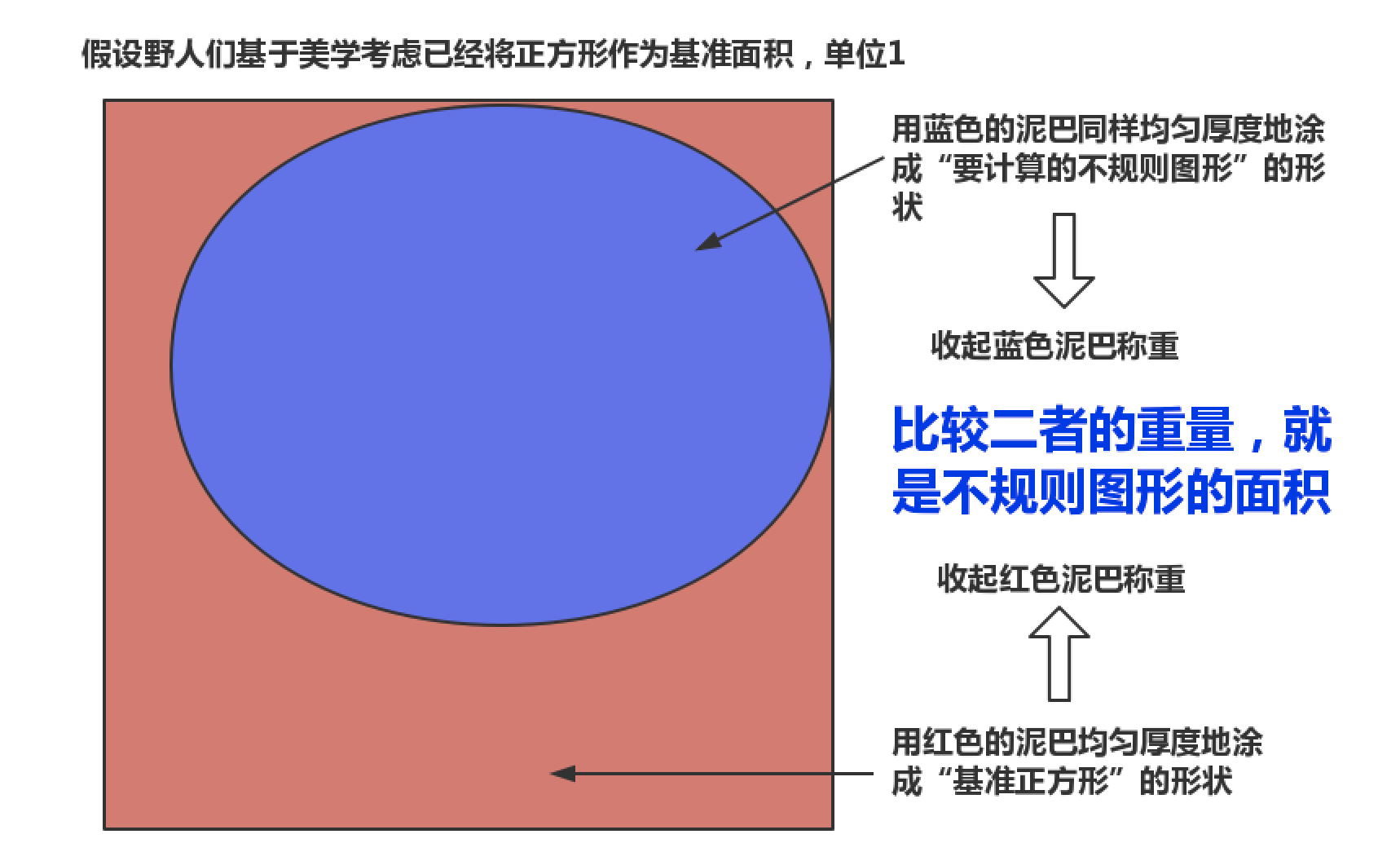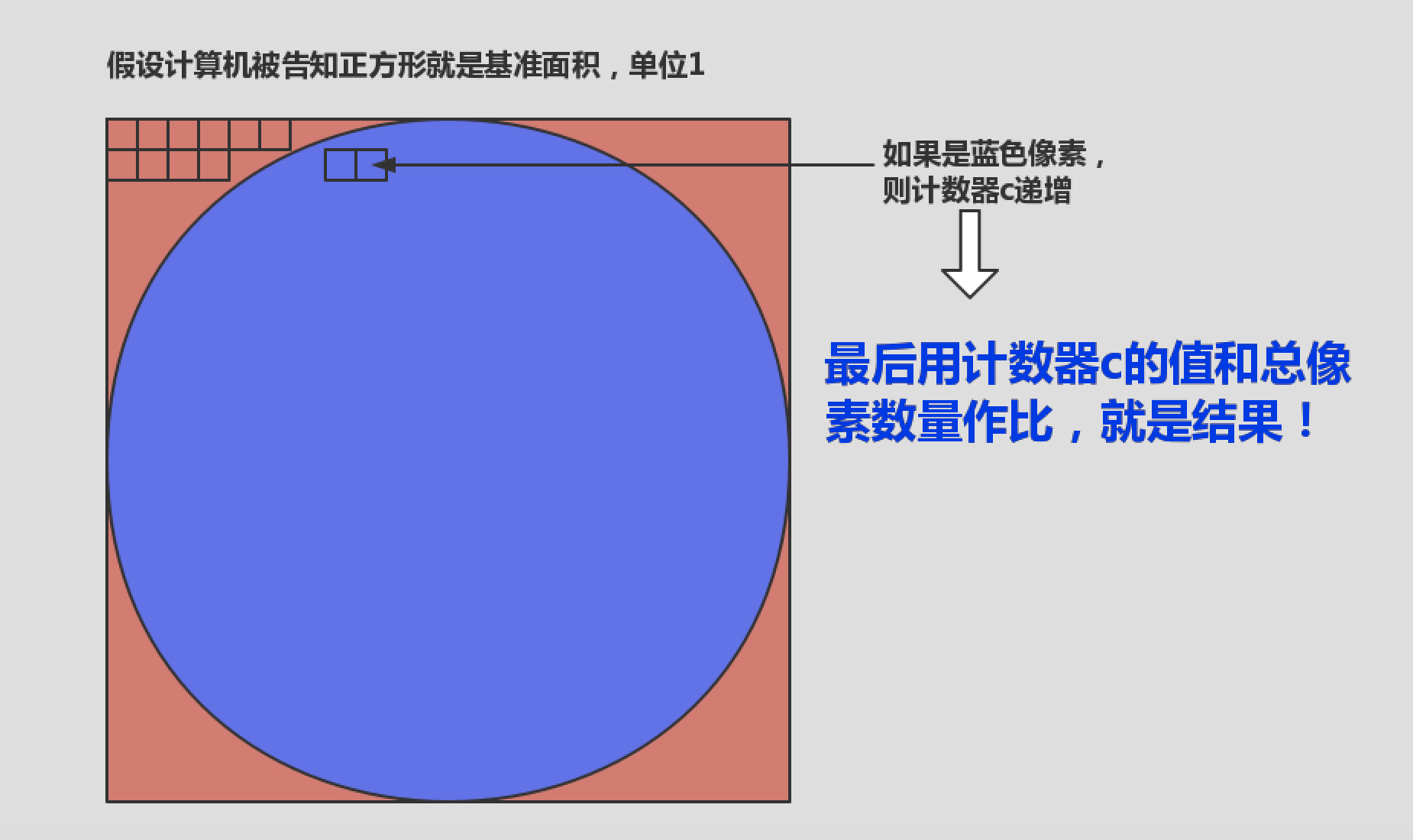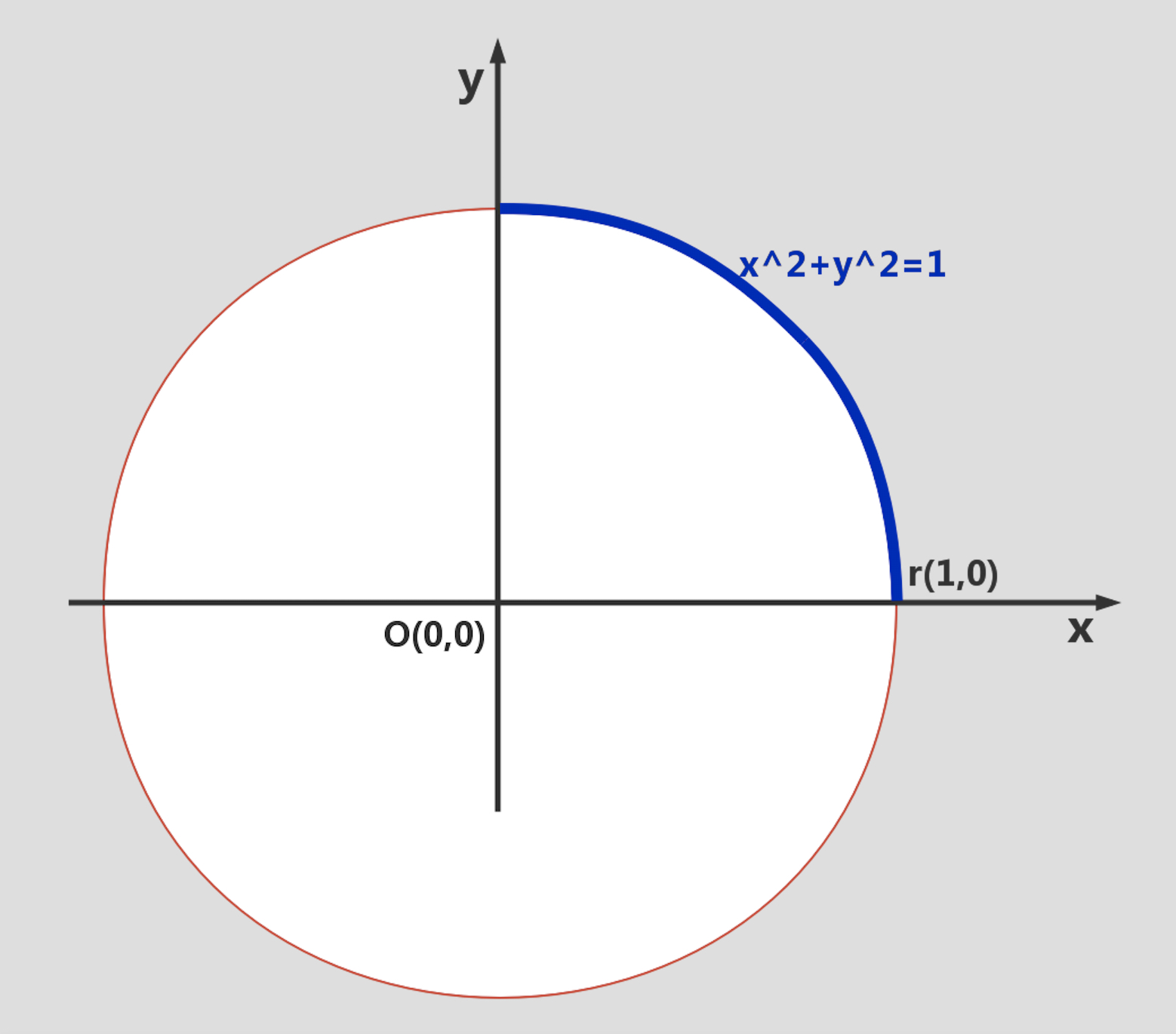# 土气和洋气的方法不用π求圆的面积• 土气的方法，即原始野人的方法
• 洋气的方法，即现代微积分的方法
• 中小学生方法，组合几何方法#include <stdio.h>
#include <stdlib.h>
#include <fcntl.h>

int main(int argc, char **argv)
{
unsigned int x, y;
int c = 0;
// l控制精度
int l = atoi(argv);
int p = l;
double k;
FILE *fp = fopen("/dev/urandom", "r");

while (l --) {
// 随机产生点的x坐标，限制在边长1000的单位正方形内
fread(&x, sizeof(int), 1, fp);
x = x%1000;

// 随机产生点的y坐标，限制在边长1000的单位正方形内
fread(&y, sizeof(int), 1, fp);
y = y%1000;

// 判断是否在单位正方形的内切圆中，如果在，则计数器递增！
if ((x-500)*(x-500) + (y-500)*(y-500) < 250000|| (x-500)*(x-500) + (y-500)*(y-500) == 250000)
c ++;
}
fclose(fp);

k = (double)c/(double)p;
printf("%d   %f\n", c, k);
}


$s=\dfrac{\pi \times 0.5^2}{1}=3.1415926\times 0.25=0.78539815$

[root@localhost rand]# ./a.out 10
5   0.500000
[root@localhost rand]# ./a.out 100
78   0.780000
[root@localhost rand]# ./a.out 1000
765   0.765000
[root@localhost rand]# ./a.out 10000
7784   0.778400
[root@localhost rand]# ./a.out 100000
78554   0.785540
[root@localhost rand]# ./a.out 1000000
784448   0.784448
[root@localhost rand]# ./a.out 10000000
7853091   0.785309
[root@localhost rand]#$S=4\times\displaystyle\int_0^1\sqrt{1-x^2}dx$

$x=\sin t$

$dx=\cos t dt$

$S=4\times \displaystyle\int_{}^{}\sqrt{1-\sin^2 t}\cos t dt=4\times \displaystyle\int_{0}^{\frac{\pi}{2}}\cos^2 t dt$

$S=4\times \dfrac{1}{2}\times\displaystyle\int_{0}^{\frac{\pi}{2}}(1+\cos 2t) dt$
$S=2\times ([t]_0^{\frac{\pi}{2}}+[\dfrac{1}{2}\sin 2t]_0^{\frac{\pi}{2}})=\pi$